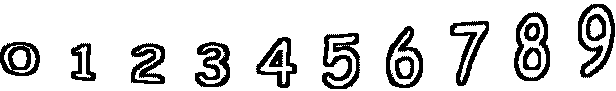5 + 3 =        4 + 1 =6 + 3 =        8 + 2 =        5 + 5 =        2 + 7 = 5 + 9 =        0 + 1 =        10 + 0 =        3 + 7 =